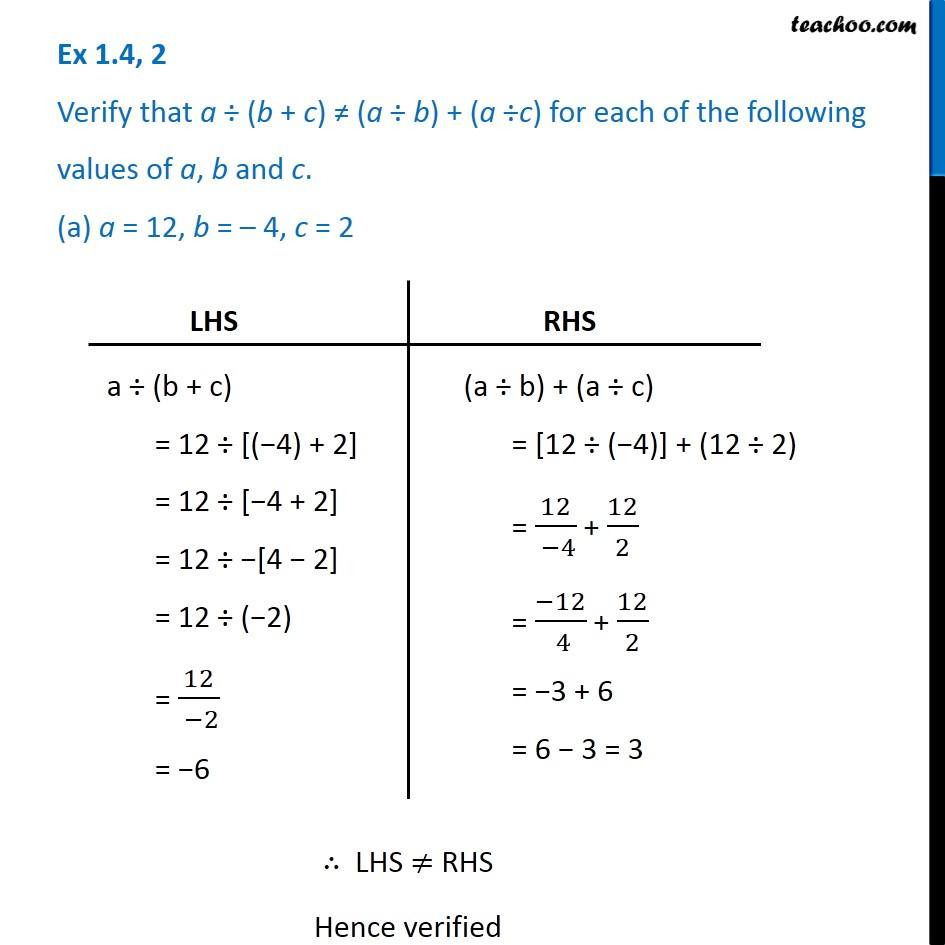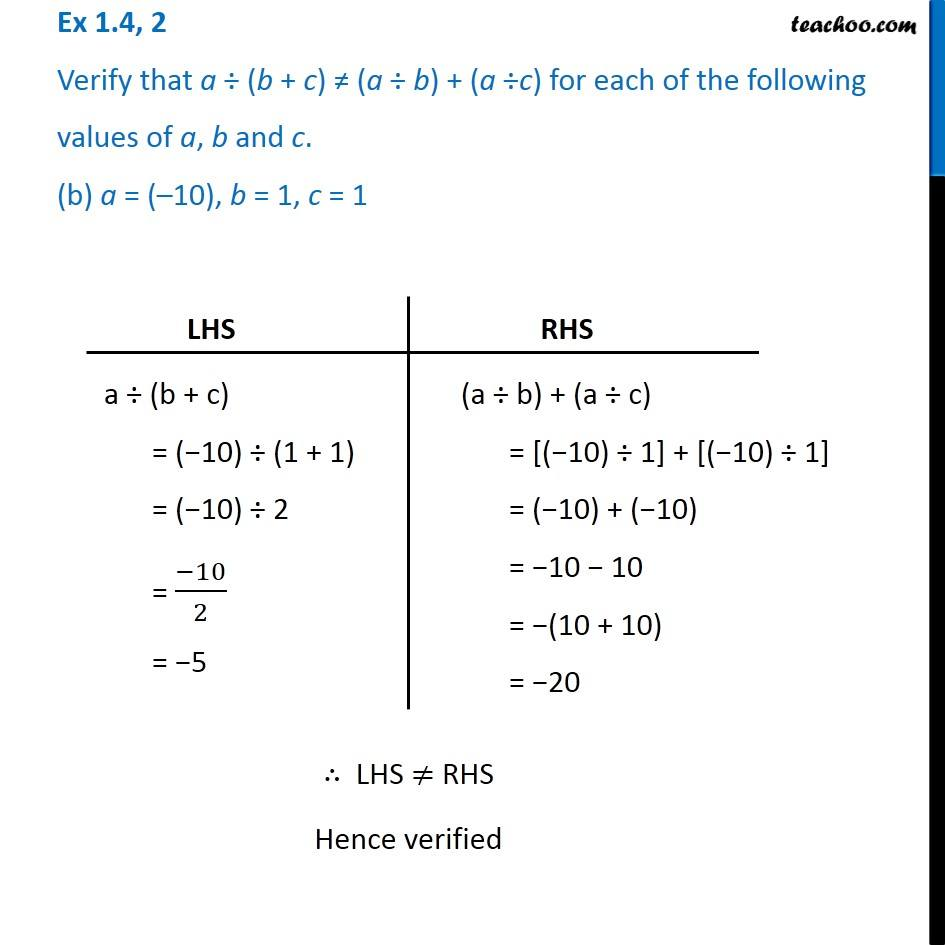1. Chapter 1 Class 7 Integers
2. Concept wise
3. Division of Integers

Transcript

Ex 1.4, 2 Verify that a (b + c) (a b) + (a c) for each of the following values of a, b and c. (a) a = 12, b = 4, c = 2 LHS a (b + c) = 12 [( 4) + 2] = 12 [ 4 + 2] = 12 [4 2] = 12 ( 2) = 12/( 2) = 6 RHS (a b) + (a c) = [12 ( 4)] + (12 2) = 12/( 4) + 12/2 = ( 12)/( 4) + 12/2 = 3 + 6 = 6 3 = 3 LHS RHS Hence verified Ex 1.4, 2 Verify that a (b + c) (a b) + (a c) for each of the following values of a, b and c. (b) a = ( 10), b = 1, c = 1 LHS a (b + c) = ( 10) (1 + 1) = ( 10) 2 = ( 10)/2 = 5 RHS (a b) + (a c) = [( 10) 1] + [( 10) 1] = ( 10) + ( 10) = 10 10 = (10 + 10) = 20 LHS RHS Hence verified

Division of Integers